Test: Random Variables

# Test: Random Variables - Electronics and Communication Engineering (ECE)

Test Description

## 20 Questions MCQ Test GATE ECE (Electronics) 2023 Mock Test Series - Test: Random Variables

Test: Random Variables for Electronics and Communication Engineering (ECE) 2023 is part of GATE ECE (Electronics) 2023 Mock Test Series preparation. The Test: Random Variables questions and answers have been prepared according to the Electronics and Communication Engineering (ECE) exam syllabus.The Test: Random Variables MCQs are made for Electronics and Communication Engineering (ECE) 2023 Exam. Find important definitions, questions, notes, meanings, examples, exercises, MCQs and online tests for Test: Random Variables below.
Solutions of Test: Random Variables questions in English are available as part of our GATE ECE (Electronics) 2023 Mock Test Series for Electronics and Communication Engineering (ECE) & Test: Random Variables solutions in Hindi for GATE ECE (Electronics) 2023 Mock Test Series course. Download more important topics, notes, lectures and mock test series for Electronics and Communication Engineering (ECE) Exam by signing up for free. Attempt Test: Random Variables | 20 questions in 60 minutes | Mock test for Electronics and Communication Engineering (ECE) preparation | Free important questions MCQ to study GATE ECE (Electronics) 2023 Mock Test Series for Electronics and Communication Engineering (ECE) Exam | Download free PDF with solutions
 1 Crore+ students have signed up on EduRev. Have you?
Test: Random Variables - Question 1

### The number of cars arriving at ICICI bank drive-in window during 10-min period is Poisson random variable X with b = 2. Que : The probability that more than 3 cars will arrive during any 10 min period is

Detailed Solution for Test: Random Variables - Question 1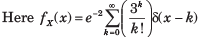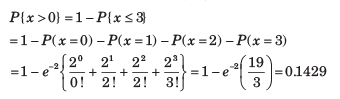Test: Random Variables - Question 2

### The number of cars arriving at ICICI bank drive-in window during 10-min period is Poisson random variable X with b = 2. Que: The probability that no car will arrive is

Detailed Solution for Test: Random Variables - Question 2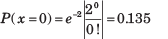Test: Random Variables - Question 3

### The power reflected from an aircraft of complicated shape that is received by a radar can be described by an exponential random variable W. The density of W is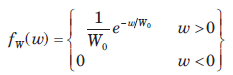where W0 is the average amount of received power. The probability that the received power is larger than the power received on the average is

Detailed Solution for Test: Random Variables - Question 3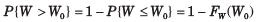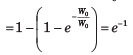Test: Random Variables - Question 4

Delhi averages three murder per week and their occurrences follow a poission distribution

Que: The probability that there will be five or more murder in a given week is

Detailed Solution for Test: Random Variables - Question 4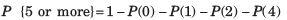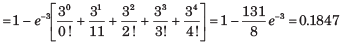Test: Random Variables - Question 5

Delhi averages three murder per week and their occurrences follow a poission distribution

Que: On the average, how many weeks a year can Delhi expect to have no murders ?

Detailed Solution for Test: Random Variables - Question 5

average number of week, per year with no murder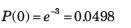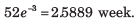Test: Random Variables - Question 6

Delhi averages three murder per week and their occurrences follow a poission distribution.

Que:How many weeds per year (average) can the Delhi expect the number of murders per week to equal orexceed the average number per week ?

Detailed Solution for Test: Random Variables - Question 6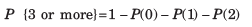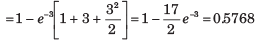Average number of weeks per year that number of murder exceeds the average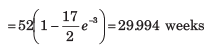Test: Random Variables - Question 7

A discrete random variable X has possible values x i = i2  i =1, 2, 3, 4 which occur with probabilities 0.4, 0.25, 0.15, 0.1,. The mean value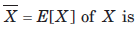Detailed Solution for Test: Random Variables - Question 7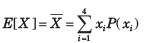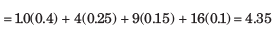Test: Random Variables - Question 8

The random variable X is defined by the density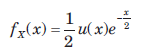The expected value of g(X)  = X3 is

Detailed Solution for Test: Random Variables - Question 8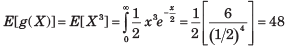Test: Random Variables - Question 9

The random variables X and Y have variances 0.2 and 0.5 respectively. Let Z= 5X-2Y. The variance of Z is?

Detailed Solution for Test: Random Variables - Question 9

Var(X) = 0.2, Var(Y) = 0.5
Z = 5X – 2Y
Var(Z) = Var(5X-2Y)
= Var(5X) + Var(2Y)
= 25Var(X) + 4Var(Y)
Var(Z) = 7.

Test: Random Variables - Question 10

The variance of random variable X is

Detailed Solution for Test: Random Variables - Question 10

Solution=

Variance of X is σx2=E[X2]- μx2

E(X2)=-∞ x2fx(x)dx=01  x2(x)3(1-x)2dx=1/10

σx2 =(1/10)-(1/4)2=3/80

hence option B would be the correct answer.

Test: Random Variables - Question 11

A Random variable X is uniformly distributed on the interval (-5, 15). Another random variableY =  e -X/5 is formed. The value of E[Y ] is

Detailed Solution for Test: Random Variables - Question 11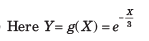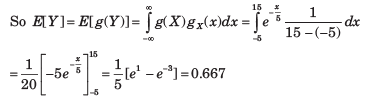Test: Random Variables - Question 12

A joint sample space for two random variable X and Y has four elements (1, 1), (2, 2), (3, 3) and (4, 4). Probabilities of these elements are 0.1, 0.35, 0.05 and 0.5 respectively.

Que: The probability of the event {X ≤ 2.5, Y ≤ 6} is

Detailed Solution for Test: Random Variables - Question 12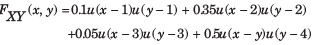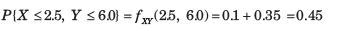Test: Random Variables - Question 13

A joint sample space for two random variable X and Y has four elements (1, 1), (2, 2), (3, 3) and (4, 4). Probabilities of these elements are 0.1, 0.35, 0.05 and 0.5 respectively.

Que: The probability of the event {X ≤ 3} is

Detailed Solution for Test: Random Variables - Question 13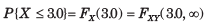= 0.1+ 0.35 + 0.05 = 0.5

Test: Random Variables - Question 14

The statistically independent random variable X and Y have mean values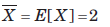.and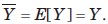They have second moments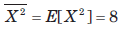and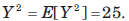Consider a random variable W = 3X - Y.

Que: The mean value E [W] is

Detailed Solution for Test: Random Variables - Question 14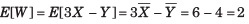Test: Random Variables - Question 15

The statistically independent random variable X and Y have mean values.andThey have second momentsandConsider a random variable W = 3X - Y.

Que: The second moment of W is

Detailed Solution for Test: Random Variables - Question 15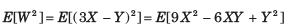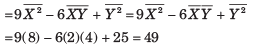Test: Random Variables - Question 16

The statistically independent random variable X and Y have mean values.andThey have second momentsandConsider a random variable W = 3X - Y.

Que: The variance of the random variable is

Detailed Solution for Test: Random Variables - Question 16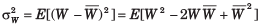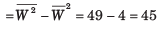Test: Random Variables - Question 17

Two random variable X and Y have the density function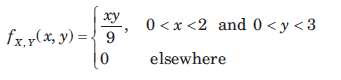The X and Y are

Detailed Solution for Test: Random Variables - Question 17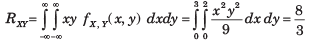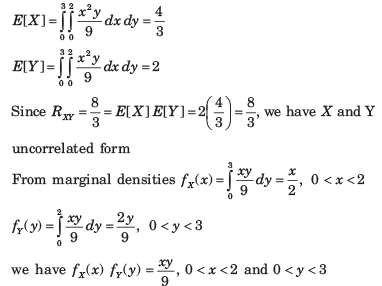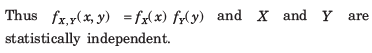Test: Random Variables - Question 18

The value of σx2 , σy2 , RXY and ρ are respectively

Detailed Solution for Test: Random Variables - Question 18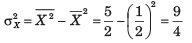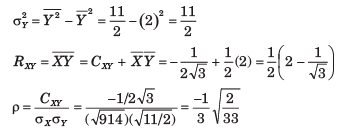Test: Random Variables - Question 19

The mean value of the random variable

W = (X + 3Y)2  + 2X + 3  is

Detailed Solution for Test: Random Variables - Question 19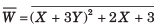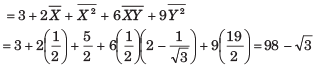Test: Random Variables - Question 20

If machine is not properly adjusted, the product resistance change to the case where ax = 1050Ω . Now the rejected fraction is

Detailed Solution for Test: Random Variables - Question 20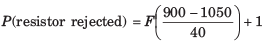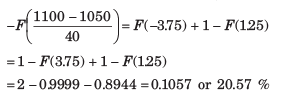## GATE ECE (Electronics) 2023 Mock Test Series

21 docs|263 tests
Information about Test: Random Variables Page
In this test you can find the Exam questions for Test: Random Variables solved & explained in the simplest way possible. Besides giving Questions and answers for Test: Random Variables, EduRev gives you an ample number of Online tests for practice

## GATE ECE (Electronics) 2023 Mock Test Series

21 docs|263 tests(Scan QR code)